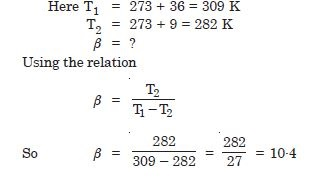# NCERT Solutions for Class 11 Physics Chapter 12 - Thermodynamics

##### Question 1:

A geyser heats water flowing at the rate of 3·0 litres per minute from 27$°$C to 77$°$C. If the geyser operates on a gas burner, what is the rate of consumption of the fuel if its heat of combustion is 40 × 104 J/g ?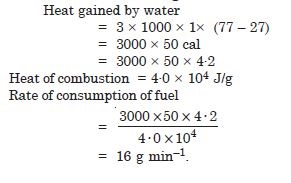##### Question 2:

What amount of heat must be supplied to 2 × 10–2 kg of nitrogen (at room temperature) to raise its temperature by 45°, at constant pressure (Molecular mass of N2 = 2.8 R = 8·3 J. mol–1).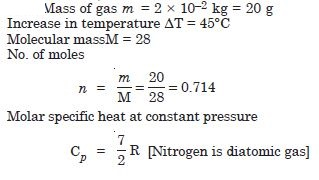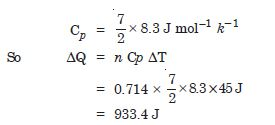##### Question 3:

Explain why
Two bodies at different temperatures T1 and T2, if brought in thermal contact do not necessarily settle to the mean temperature (T1 + T2)/2.

The temperature of both the bodies will not be mean. It depends upon the conductivity and specific heat of the body.

##### Question 4:

Explain why
The coolant in a chemical or a nuclear plant (i.e., the liquid used to prevent the different parts of a plant from getting too hot) should have high specific heat ?

If the specific heat is large, it can absorb large amount of heat, before it starts boiling.

##### Question 5:

Explain why
Air pressure in a car tyre increases during driving.

Due to increase in temp. pressure increases.

##### Question 6:

A cylinder with a movable piston contains 3 moles of hydrogen at standard temperature and pressure. The walls of the cylinder are made of a heat insulate and the piston is insulated by having a pile of sand on it. By what factor does pressure of gas increase if the gas is compressive to half its original volume ?##### Question 7:

In changing the state of a gas adiabatically from an equilibrium state A to another equilibrium state B, an amount of work equal to 22.3 J is done on the system. If the gas taken from state A to B via a process in which net heat absorbed by the system is 9.35 cal, how much is the net work done by the system in latter case (take 1 cal = 4·19 J)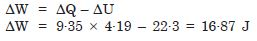##### Question 8:

Two cylinders A and B of equal capacity are connected to each other via a stopcock. A contains a gas at standard temperature and pressure. B is completely evacuated. The entire system is thermally insulated. The stopcock is suddenly opened.
What is the final pressure of the gas in A and B ?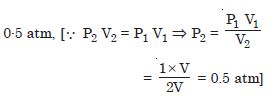##### Question 9:

Two cylinders A and B of equal capacity are connected to each other via a stopcock. A contains a gas at standard temperature and pressure. B is completely evacuated. The entire system is thermally insulated. The stopcock is suddenly opened.
What is the change in internal energy of the gas ?

Zero

##### Question 10:

Two cylinders A and B of equal capacity are connected to each other via a stopcock. A contains a gas at standard temperature and pressure. B is completely evacuated. The entire system is thermally insulated. The stopcock is suddenly opened.
What is the change in the temperature of the gas ?

Zero (assuming the gas to be ideal).

##### Question 11:

Two cylinders A and B of equal capacity are connected to each other via a stopcock. A contains a gas at standard temperature and pressure. B is completely evacuated. The entire system is thermally insulated. The stopcock is suddenly opened.
Do the intermediate states of the system (before settling to the final equilibrium rate) on its P–V–T surface ?

No, since the process (called free expansion) is rapid and cannot be controlled. The intermediate states are non-equilibrium states and do not satisfy the gas equation. In due course, the gas does return to an equilibrium state, which lies on its P–V–T surface.

##### Question 12:

A steam engine delivers 5.4 × 108 J of work per minute and services 3.6 × 109 J of heat per minute from its boiler. What is the efficiency of the engine ? How much heat is wanted per minute ?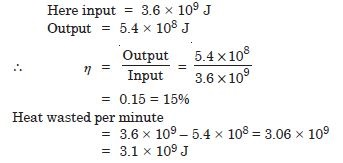##### Question 13:

An electric heater supplies heat to a system at a rate of 100 W. If system performs work at a rate of 75 joule per second. At what rate is the internal energy increasing ?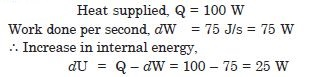##### Question 14:

A thermodynamic system is taken from an original state to an intermediate state by the linear process as shown in Fig. 12.2.
Its volume is then reduced to the original value from E to F by an isobaric process. Calculate the work done by the gas from D to E to F.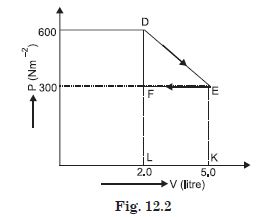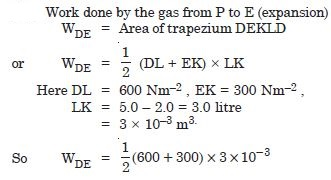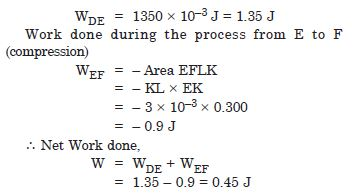A refrigerator is to remove heat from the estables kept inside at 9$°$C. Since if room temperature is 36$°$C, calculate the coefficient of performance.